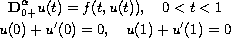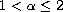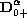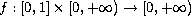Electron. J. Diff. Eqns., Vol. 2006(2006), No. 36, pp. 1-12.

### Positive solutions for boundary-value problems of nonlinear fractional differential equations Shuqin Zhang

Abstract:
In this paper, we consider the existence and multiplicity of positive solutions for the nonlinear fractional differential equation boundary-value problemwhereis a real number, andis the Caputo's fractional derivative, andis continuous. By means of a fixed-point theorem on cones, some existence and multiplicity results of positive solutions are obtained.

Submitted November 30, 2005. Published March 21, 2006.
Math Subject Classifications: 34B15.
Key Words: Caputo's fractional derivative; fractional differential equation; boundary-value problem; positive solution; fractional Green's function; fixed-point theorem

An addendum as attached on November 9, 2009. It corrects the definition of n in Lemmas 2.2 and 2.3. See the last page of this article.

Show me the PDF file (229K), TEX file, and other files for this article.Shuqin Zhang Department of Mathematics University of Mining and Technology Beijing, 100083 China email: zhangshuqin@tsinghua.org.cn